# Rounding and issuing change in multiple currencies

btw I’m wondering where @mjb2000 is. Matt did you gave up?

I have 4 Change Transactions and 4 Change Templates - 2 are using Default Currency (USD), and 2 are using Foreign Currency (HNL). However, I will probably not use the last Change Template (Credit Customer HNL) so I do not have it mapped right now.

It credits the Customer Account for 38 (USD). It displays “Credit Customer Account: 38.00”, and the Accounts screen reflects the same. But since I type “30” then USD Change button, I expected it to Credit Customer Account only 8 (USD), and have no HNL Change dispensed.

1 Like

OK. There is a problem there. I’m checking it.

Ok, tried that.

I set both Cash USD and Credit Account USD Transaction Types to USD Currency: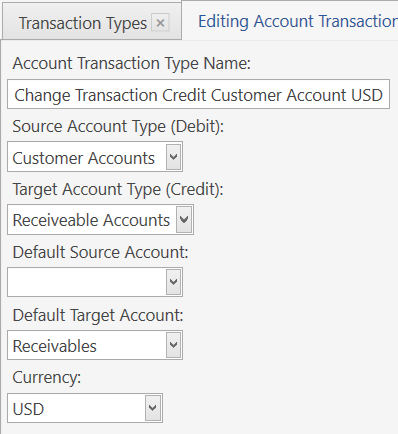It does as expected according to Accounts and Transactions:

P.S. It behaves the same way if I leave Cash USD Transaction set to `blank`. Only Credit Account needs USD Currency set.

Not too sure on the way it displays the operation (green button indicates about to click). It is the 3rd step in this sequence that is confusing to me by what it displays. Maybe the “30” amounts on the first and last buttons should be (bracketed) to indicate the value has been removed? And to be consistent, the middle button could display in the same format as the first and last button? (I sort of see why it doesn’t but…)

1 Like

Ok? LOL.

I notice there is no currency symbol for Account Credit, so I assume you “fixed” it so we don’t need to set Default Currency and can leave it blank for that Tx Type? Or have you just not defined a format for the currency (ie. `\$ {0:N}`)

Total is 6.49 and you Tendered 10?
Type “2” (shown bracketed to indicate change being paid) with remaining amount shown last (1.51)
Now click on middle button - what does it show then? That is the part that confused me.

It shows `Change Amount 3.51`. This is a silly operation as you (operator lol) starts splitting change amount but selected main currency on all steps.

[quote=“QMcKay, post:138, topic:13051”]
so I assume you “fixed” it so we don’t need to set Default Currency and can leave it blank for that Tx Type?
[/quote]Yes I fixed it.

1 Like

Looks great @emre ! Works very well.

I switched Customer Account Credit Transaction Type back to blank and deleted the USD “default” Currency.

Printing Change Amounts using these Sections - the last one handle Customer Account Credits:

``````[CHANGES:Cash USD]
<W00:27, 2, 9, 3, 7>{CHANGE PAYMENT NAME}|||\$|{CHANGE PAYMENT AMOUNT}

[CHANGES:Cash HNL]
<W00:27, 2, 9, 3, 7>{CHANGE PAYMENT NAME}|L|[=F(Math.round(TN('{EXCHANGE AMOUNT}')))]|\$|[=F('{CHANGE PAYMENT AMOUNT}')]

[CHANGES]
<W00:27, 2, 9, 3, 7>{CHANGE PAYMENT NAME}|L|[=F(Math.round(TN('{CHANGE PAYMENT AMOUNT}')/TN('{EXCHANGE RATE:HNL}')))]|\$|[=F('{CHANGE PAYMENT AMOUNT}')]
``````

So when I split the Change Amount between Cash USD and Account Credit, this is produced: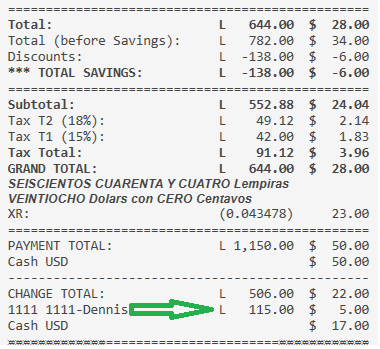It might seem silly, but my Customers do this frequently. Sometimes they pay and want Change to go on Account as Credit. Sometimes they want all Cash.

But now and then I run into this scenario: their Account is overdrawn (they owe from a previous visit), they pay and want Credit applied to the Account to settle it to `0.00` and receive the rest of the Change in Cash. That way they walk away with a clear Account and the remainder in Cash. Happens quite a bit.

For example, this video shows a Customer Account that owes \$5. So they pay the \$76 Bill by Tendering \$100 giving them \$24 Change. They use some of the Change (5) to zero their Account and take the rest of the Change in Cash (19).

@emre we have another problem again with the Rounding issue. I will keep this in the same Topic because it probably applies in the same way, but this has to do with Reporting.

I have this Report, which looks a bit scary …

``````[Cashout Report:1, 1, 1, 1, 1]
>>Tender|Count|(-Float)|Account|+/-
HNL|[=F(TN('{SETTING:HNLcountTotal}'),'0.00')]|[=F(TN('{SETTING:HNLcountTotal}')-TN('{SETTING:HNLfloat}'),'0.00')]|[=F(TN('{ACCOUNT TOTAL:Cash HNL}')*TN('{SETTING:XR_USDtoHNL}'),'0.00')]|[=F(TN('{SETTING:HNLcountTotal}')-TN('{SETTING:HNLfloat}')-(TN('{ACCOUNT TOTAL:Cash HNL}')*TN('{SETTING:XR_USDtoHNL}')),'0.00')]
USD|[=F(TN('{SETTING:USDcountTotal}'),'0.00')]|[=F(TN('{SETTING:USDcountTotal}')-TN('{SETTING:USDfloat}'),'0.00')]|[=F(TN('{ACCOUNT TOTAL:Cash USD}'),'0.00')]|[=F(TN('{SETTING:USDcountTotal}')-TN('{SETTING:USDfloat}')-TN('{ACCOUNT TOTAL:Cash USD}'),'0.00')]
CC HNL|[=F(TN('{SETTING:CCcountTotal}'),'0.00')]|-|[=F(TN('{ACCOUNT TOTAL:Credit Card HNL}'),'0.00')]|[=F(TN('{SETTING:CCcountTotal}')-TN('{ACCOUNT TOTAL:Credit Card HNL}'),'0.00')]
CC USD|[=F(TN('{SETTING:CCUSDcountTotal}'),'0.00')]|-|[=F(TN('{ACCOUNT TOTAL:Credit Card USD}'),'0.00')]|[=F(TN('{SETTING:CCUSDcountTotal}')-TN('{ACCOUNT TOTAL:Credit Card USD}'),'0.00')]
>>Account|HNL|USD|-|TTL USD
Tips HNL|[=F(TN('{ACCOUNT TOTAL:Tips}')*TN('{SETTING:XR_USDtoHNL}'))]|[=F(TN('{ACCOUNT TOTAL:Tips}'))]|-|[=F(TN('{ACCOUNT TOTAL:Tips}'))]
Round|[=F(TN('{ACCOUNT TOTAL:Rounding HNL}'))]|[=F(TN('{ACCOUNT TOTAL:Rounding USD}'))]|-|[=F(TN('{ACCOUNT TOTAL:Rounding USD}')+TN('{ACCOUNT TOTAL:Rounding HNL}'))]
>XR/TTL|[=F(TN('{SETTING:XR_USDtoHNL}'),'0.00')]|[=F(TN('{SETTING:XR_HNLtoUSD}'),'0.000000')]|-|[=F(TN('{ACCOUNT TOTAL:Tips}')+TN('{ACCOUNT TOTAL:Rounding USD}')+TN('{ACCOUNT TOTAL:Rounding HNL}'))]

>>TOTALS|Count|(-Float)|Account|+/-
HNL|[=F(TN('{SETTING:HNLcountTotal}')+TN('{SETTING:CCcountTotal}')+TN('{SETTING:CCUSDcountTotal}')*TN('{SETTING:XR_USDtoHNL}')+TN('{SETTING:USDcountTotal}')*TN('{SETTING:XR_USDtoHNL}'),'0.00')]|[=F( TN('{SETTING:HNLcountTotal}')-TN('{SETTING:HNLfloat}') + TN('{SETTING:USDcountTotal}')*TN('{SETTING:XR_USDtoHNL}')-TN('{SETTING:USDfloat}')*TN('{SETTING:XR_USDtoHNL}') + TN('{SETTING:CCcountTotal}') + TN('{SETTING:CCUSDcountTotal}')*TN('{SETTING:XR_USDtoHNL}'),'0.00')]|[=F((TN('{ACCOUNT TOTAL:Cash HNL}')+TN('{ACCOUNT TOTAL:Cash USD}')+TN('{ACCOUNT TOTAL:Credit Card HNL}')+TN('{ACCOUNT TOTAL:Credit Card USD}')+TN('{ACCOUNT TOTAL:Tips}'))*TN('{SETTING:XR_USDtoHNL}'),'0.00')]|[=F(TN('{SETTING:HNLcountTotal}')-TN('{SETTING:HNLfloat}') + TN('{SETTING:USDcountTotal}')*TN('{SETTING:XR_USDtoHNL}')-TN('{SETTING:USDfloat}')*TN('{SETTING:XR_USDtoHNL}') + TN('{SETTING:CCcountTotal}') + TN('{SETTING:CCUSDcountTotal}')*TN('{SETTING:XR_USDtoHNL}') - ((TN('{ACCOUNT TOTAL:Cash HNL}')+TN('{ACCOUNT TOTAL:Cash USD}')+TN('{ACCOUNT TOTAL:Credit Card HNL}')+TN('{ACCOUNT TOTAL:Credit Card USD}')+TN('{ACCOUNT TOTAL:Tips}')))*TN('{SETTING:XR_USDtoHNL}'),'0.00')]
USD|[=F(((TN('{SETTING:HNLcountTotal}')+TN('{SETTING:CCcountTotal}'))/TN('{SETTING:XR_USDtoHNL}'))+TN('{SETTING:CCUSDcountTotal}')+TN('{SETTING:USDcountTotal}'),'0.00')]|[=F( ((TN('{SETTING:HNLcountTotal}')-TN('{SETTING:HNLfloat}'))/TN('{SETTING:XR_USDtoHNL}')) + TN('{SETTING:USDcountTotal}')-TN('{SETTING:USDfloat}') + (TN('{SETTING:CCcountTotal}')/TN('{SETTING:XR_USDtoHNL}')) + TN('{SETTING:CCUSDcountTotal}'),'0.00')]|[=F(TN('{ACCOUNT TOTAL:Cash HNL}')+TN('{ACCOUNT TOTAL:Cash USD}')+TN('{ACCOUNT TOTAL:Credit Card HNL}')+TN('{ACCOUNT TOTAL:Credit Card USD}')+TN('{ACCOUNT TOTAL:Tips}'),'0.00')]|[=F(TN('{SETTING:HNLcountTotal}')/TN('{SETTING:XR_USDtoHNL}')-TN('{SETTING:HNLfloat}')/TN('{SETTING:XR_USDtoHNL}') + TN('{SETTING:USDcountTotal}')-TN('{SETTING:USDfloat}') + TN('{SETTING:CCcountTotal}')/TN('{SETTING:XR_USDtoHNL}') + TN('{SETTING:CCUSDcountTotal}') - (TN('{ACCOUNT TOTAL:Cash HNL}')+TN('{ACCOUNT TOTAL:Cash USD}')+TN('{ACCOUNT TOTAL:Credit Card HNL}')+TN('{ACCOUNT TOTAL:Credit Card USD}')+TN('{ACCOUNT TOTAL:Tips}')),'0.00')]
``````

Even though the Report looks a bit daunting, It produces this nice Cashout Count Report for reconciling Cash in Drawer Counts and compares that to values in Accounts so we can see if we are over or short in Cash. The problem is outlined in RED …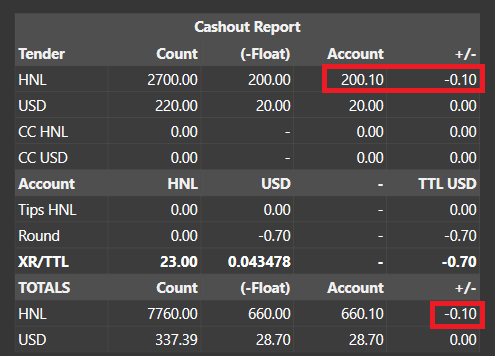To narrow it down, there is this expression in there, which should tell me how much HNL Cash is in the Account (which should also be in my drawer):

``````[=F(TN('{ACCOUNT TOTAL:Cash HNL}')*TN('{SETTING:XR_USDtoHNL}'),'0.00')]
... which could also be expressed using inverted rate as ...
[=F(TN('{ACCOUNT TOTAL:Cash HNL}')/TN('{SETTING:XR_HNLtoUSD}'),'0.00')]
``````

NOTE:
`{SETTING:XR_USDtoHNL}` contains 23
`{SETTING:XR_HNLtoUSD}` contains 0.0434782608695652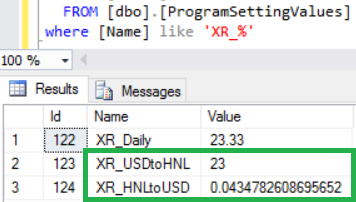Either way …
That line is returning 200.10 … that is the problem.
I know that I took in HNL 200.00.
At XR 23 that comes to: USD 8.695652 - and that is how it is shown in the DB - so far so good: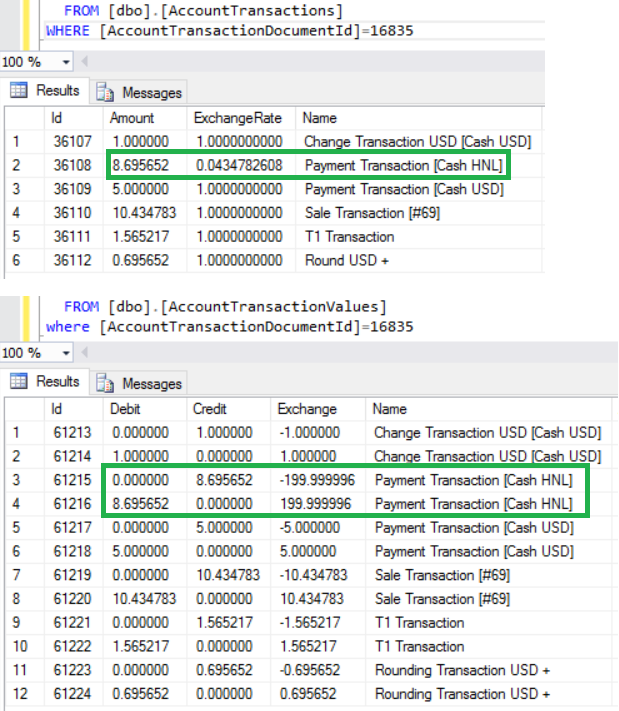The problem is that the Report Tag for `{ACCOUNT TOTAL:X}` is returning the Rounded value of USD `8.70`
When that is converted at XR 23, it comes out as HNL `200.10`
So it makes reconciliation confusing to the operator because of the `0.10` difference being reported.

@QMcKay thank you for reporting it. I changed account tags to printing currency format and uploaded another update. Please let me know if it solves the issue or not.

1 Like

Ok thank you - will test right away, and let you know very soon.

I quickly started SQL route this morning, but it will be much more involved and messy compared to the relatively straight-forward and simple Report Tags like `{ACCOUNT TOTAL:X}`.

Transactions for Account:

``````SELECT
tv.[Id]
,[Debit]
,[Credit]
,[Exchange]
,tv.[Name]
,act.[Name] as [AccountType]
,ac.[Name] as [AccountName]
,actt.[Name] as [TxType]
--,[Date]
--,[AccountTransactionDocumentId]
--,[AccountTransactionId]
--,tv.[AccountTypeId]
--,[AccountId]
--,[AccountTransactionTypeId]
--,[DepartmentId]
FROM [AccountTransactionValues] tv
JOIN [AccountTypes] act on act.[Id]=tv.[AccountTypeId]
JOIN [Accounts] ac on ac.[Id]=tv.[AccountId]
JOIN [AccountTransactionTypes] actt on actt.[Id]=tv.[AccountTransactionTypeId]
WHERE 1=1
--AND tv.[Name] like '%HNL%'
AND ac.[Name] = 'Cash HNL'
ORDER BY [AccountTransactionDocumentId],[Date]--,[AccountTransactionId]
``````

Account Balance:

``````DECLARE @StartDate datetime = '2017-01-02 21:00:00'
SELECT
sum([Debit]) as [Debit]
,sum([Credit]) as [Credit]
,sum([Exchange]) as [Exchange]
,ac.[Name] as [AccountName]
FROM [AccountTransactionValues] tv
JOIN [Accounts] ac on ac.[Id]=tv.[AccountId]
WHERE 1=1
AND ac.[Name] = 'Cash HNL'
AND tv.[Date] >= @StartDate
GROUP BY ac.[Name]
``````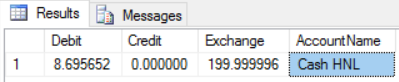Account Balances for ALL Accounts from beginning of time:

``````DECLARE @StartDate datetime = '2017-01-02 21:00:00'
SELECT
sum([Debit]) as [Debit]
,sum([Credit]) as [Credit]
,sum([Exchange]) as [Exchange]
,act.[Name] as [AccountType]
,ac.[Name] as [AccountName]
FROM [AccountTransactionValues] tv
JOIN [Accounts] ac on ac.[Id]=tv.[AccountId]
JOIN [AccountTypes] act on act.[Id]=tv.[AccountTypeId]
WHERE 1=1
--AND act.[Name] IN ('Payment Accounts','Customer Accounts')
--AND ac.[Name] IN ('Cash HNL','Cash USD','Credit Card HNL','Credit Card USD')
--AND tv.[Date] >= @StartDate
GROUP BY ac.[Name],act.[Name]
``````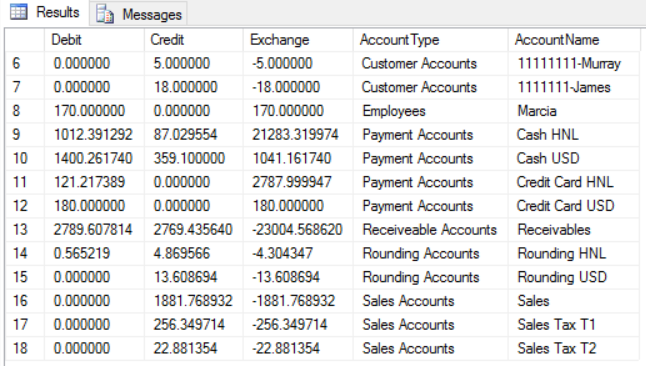@emre, I think it would be helpful if you could also expose `{EXCHANGE RATE:X}` to Report Tags. Would save needing to store the XR in a Program Setting.

Initial tests look Good!Missed oneHi guys, no I didn’t give up, but for the last 3 weeks we’ve been in high season and went from 8 divers per day to around 40… It’s been very busy with lots of late nights and no time to touch SambaPOS. I’m back on it now and trying to get my head around the last few things, including reports…

I will have a proper read through everything over the next couple of days and see where I’m up toOne question I have, is how I can I customise the thresholds for whcih direction the rounding goes? I described this is a little it more in this post:

Happy new year!

Sorry to bring this back from the dead, @QMcKay, did you ever get to the bottom of this?

I was using Payment total - Ticket total but when I print a ticket prior to payment (for a customer to review the proposed charges), this calculation clearly does not work for the intended purpose.

I haven’t tested it lately, but assume it is still “broken”.

What do you mean by payment total - ticket total does not work?

EDIT: P.S. This is what I use - notice there are 3 lines wrapped inside square brackets `[...]` which makes printing conditional, meaning that if there is no Change Due, nothing prints in that space :

``````<W00:28, 10, 10>XR:|([=F('{EXCHANGE RATE:HNL}','0.000000')])|[=F(1/TN('{EXCHANGE RATE:HNL}'))]
<F>-
-- <L00>CHANGE:{CHANGE TOTAL}
[<W00:27, 2, 9, 3, 7>[=(TN('{PAYMENT TOTAL}')-TN('{TICKET TOTAL}')) > 0 ? 'CHANGE TOTAL:|L|' + F((TN('{PAYMENT TOTAL}')-TN('{TICKET TOTAL}'))/TN('{EXCHANGE RATE:HNL}')) +'|\$|'+ F(TN('{PAYMENT TOTAL}')-TN('{TICKET TOTAL}')) : '']
{CHANGES}
<F>=]
<EB>
<T>Tip/Propina
<C00>Suggested Amounts NOT INCLUDED!
<DB>
``````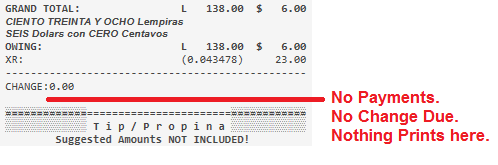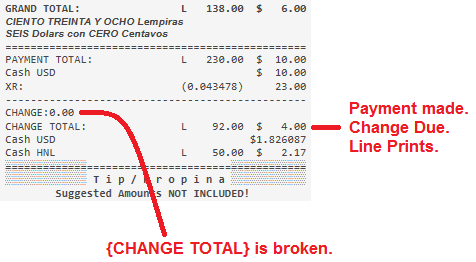@emre, `{CHANGE TOTAL}` is still broken in multi-currency setup for some reason. Here is my full latest Template:

``````[LAYOUT]
-- line char count is 48
<EC>
<XCT>29,40,76,6,0,48,69,32,32,1,1
<DC>
<J00> |
<EB>
<DB>
<W00:31,10, 7>{TICKET DATE} {TICKET TIME}|Server: {USER NAME}|#{TICKET NO}
{ENTITIES}
[=(('{ENTITY DATA:Customers:RTN}' == '') && ('{TICKET TAG:BUS_RTN}' == '')) ? '<C00>Consumidor Final' : '']
[<J00> |
<L00>BUS:[=('{ENTITY DATA:Customers:Business}' == '' ? ('{TICKET TAG:BUS_NM}' == '' ? '___________________________________________' : '{TICKET TAG:BUS_NM}') : '{ENTITY DATA:Customers:Business}')]
<J00> |
<L00>RTN:[=('{ENTITY DATA:Customers:RTN}' == '' ? ('{TICKET TAG:BUS_RTN}' == '' ? '___________________________________________' : '{TICKET TAG:BUS_RTN}') : '{ENTITY DATA:Customers:RTN}')]]
<EB>
[<L00>NOTE: [{NOTE}]]
<DB>
<F>-
{ORDERS}
<F>=
<EB>
<W00:27, 2, 9, 3, 7>Total:|L|[=F((TN('{TICKET TOTAL}')-TN('{CALCULATION TOTAL: }'))/TN('{EXCHANGE RATE:HNL}'))]|\$|[=F(TN('{TICKET TOTAL}')-TN('{CALCULATION TOTAL: }'))]
<DB>
[<W00:27, 2, 9, 3, 7>Total (before Savings):|L|[=F( ( TN('{TICKET TOTAL}')-TN('{CALCULATION TOTAL: }') + ( (TN('{ORDER STATE TOTAL:Gift}'))+(-1*TN('{ORDER TAG TOTAL:Discount USD}'))+(-1*TN('{ORDER TAG TOTAL:VIP Discount}'))+(-1*TN('{ORDER TAG TOTAL:HH Discount}'))+(-1*TN('{CALCULATION TOTAL:Discount}')) ) )/TN('{EXCHANGE RATE:HNL}') )]|\$|[=F( ( TN('{TICKET TOTAL}')-TN('{CALCULATION TOTAL: }') + ( (TN('{ORDER STATE TOTAL:Gift}'))+(-1*TN('{ORDER TAG TOTAL:Discount USD}'))+(-1*TN('{ORDER TAG TOTAL:VIP Discount}'))+(-1*TN('{ORDER TAG TOTAL:HH Discount}'))+(-1*TN('{CALCULATION TOTAL:Discount}')) ) ) )]]
{DISCOUNTS}
[<W00:27, 2, 9, 3, 7>Discounts:|L|-[=F((-1*TN('{ORDER TAG TOTAL:Discount USD}')+(-1*(TN('{ORDER TAG TOTAL:VIP Discount}')+TN('{ORDER TAG TOTAL:HH Discount}')+(-1*TN('{CALCULATION TOTAL:Discount}')))))/TN('{EXCHANGE RATE:HNL}'))]|\$|-[=F(-1*TN('{ORDER TAG TOTAL:Discount USD}')+(-1*(TN('{ORDER TAG TOTAL:VIP Discount}')+TN('{ORDER TAG TOTAL:HH Discount}')+(-1*TN('{CALCULATION TOTAL:Discount}')))))]]
<EB>
[<W00:27, 2, 9, 3, 7>*** TOTAL SAVINGS:|L|-[=F(((-1*TN('{ORDER TAG TOTAL:Discount USD}')+(-1*(TN('{ORDER TAG TOTAL:VIP Discount}')+TN('{ORDER TAG TOTAL:HH Discount}')))+TN('{ORDER STATE TOTAL:Gift}')+(-1*TN('{CALCULATION TOTAL:Discount}'))))/TN('{EXCHANGE RATE:HNL}'))]|\$|-[=F((-1*TN('{ORDER TAG TOTAL:Discount USD}')+(-1*(TN('{ORDER TAG TOTAL:VIP Discount}')+TN('{ORDER TAG TOTAL:HH Discount}')))+TN('{ORDER STATE TOTAL:Gift}')+(-1*TN('{CALCULATION TOTAL:Discount}'))))]]
<DB>
<F>=
<EB>
<W00:27, 2, 9, 3, 7>Subtotal:|L|[=F((TN('{TICKET TOTAL}')-TN('{TAX TOTAL}')-TN('{CALCULATION TOTAL:Round HNL +}'))/TN('{EXCHANGE RATE:HNL}'))]|\$|[=(F(TN('{TICKET TOTAL}')-TN('{TAX TOTAL}')-TN('{CALCULATION TOTAL:Round HNL +}')))]
<DB>
{TAXES}
<EB>
<W00:27, 2, 9, 3, 7>Tax Total:|L|[=F(TN('{TAX TOTAL}')/TN('{EXCHANGE RATE:HNL}'))]|\$|[=F('{TAX TOTAL}')]
<DB>
{SERVICES}
<EB>
<W10:27, 2, 9, 3, 7>GRAND TOTAL:|L|[=F(Math.round(TN('{TICKET TOTAL}')/TN('{EXCHANGE RATE:HNL}')))]|\$|[=F(TN('{TICKET TOTAL}'))]
<DB>
<span style="font-family:Arial;font-size:10;font-style:italic;font-weight:bold;">{CALL:num.numToSpanish('{TICKET TOTAL}','{EXCHANGE RATE:HNL}')} Lempiras</span>
<span style="font-family:Arial;font-size:10;font-style:italic;font-weight:bold;">{CALL:num.numToSpanish('{TICKET TOTAL}',1)} Dolars con {CALL:num.numToSpanish('{TICKET TOTAL}'.substr('{TICKET TOTAL}'.indexOf('.')+1)/1000,1)} Centavos</span>
[<F>=
<W00:27, 2, 9, 3, 7>PAYMENT TOTAL:|L|[=F(TN('{PAYMENT TOTAL}')/TN('{EXCHANGE RATE:HNL}'))]|\$|[=F(TN('{PAYMENT TOTAL}'))]]
{PAYMENTS}
<EB>
[=(TN('{TICKET TOTAL}')-TN('{PAYMENT TOTAL}'))>0 ? '<W10:27, 2, 9, 3, 7>OWING:|L|' +F(Math.round((TN('{TICKET TOTAL}')-TN('{PAYMENT TOTAL}'))/TN('{EXCHANGE RATE:HNL}'))) + '|\$|' +F(TN('{TICKET TOTAL}')-TN('{PAYMENT TOTAL}')) : '']
<DB>
[<L00>CC Info: {TICKET TAG:CCtype} ({TICKET TAG:CCdigits})]
<W00:28, 10, 10>XR:|([=F('{EXCHANGE RATE:HNL}','0.000000')])|[=F(1/TN('{EXCHANGE RATE:HNL}'))]
<F>-
<L00>CHANGE:{CHANGE TOTAL}
[<W00:27, 2, 9, 3, 7>[=(TN('{PAYMENT TOTAL}')-TN('{TICKET TOTAL}')) > 0 ? 'CHANGE TOTAL:|L|' + F((TN('{PAYMENT TOTAL}')-TN('{TICKET TOTAL}'))/TN('{EXCHANGE RATE:HNL}')) +'|\$|'+ F(TN('{PAYMENT TOTAL}')-TN('{TICKET TOTAL}')) : '']
{CHANGES}
<F>=]
<EB>
<T>Tip/Propina
<C00>Suggested Amounts NOT INCLUDED!
<DB>
<W00:27, 2, 9, 3, 7>10%:|L|[=F(TN('{TICKET TOTAL}')/{EXCHANGE RATE:HNL} * 0.10)]|\$|[=F(TN('{TICKET TOTAL}') * 0.10)]
<W00:27, 2, 9, 3, 7>15%:|L|[=F(TN('{TICKET TOTAL}')/{EXCHANGE RATE:HNL} * 0.15)]|\$|[=F(TN('{TICKET TOTAL}') * 0.15)]
<W00:27, 2, 9, 3, 7>20%:|L|[=F(TN('{TICKET TOTAL}')/{EXCHANGE RATE:HNL} * 0.20)]|\$|[=F(TN('{TICKET TOTAL}') * 0.20)]
<EB>
<C00>ALL TIPS go to our Awesome Staff!
<DB>
[<F>=
<J00>{TICKET TAG:GCN}|Balance: [=F(TN('{TICKET TAG:GCB}'))]]
<EB>
<F>*
<L00>*               HAPPY HOUR 5-7pm               *
<F>*
<L00>*                  {SETTING:DEI_PHONE}                   *
<L00>*              {SETTING:DEI_WEB}              *
<L00>*             {SETTING:DEI_EMAIL}              *
<F>*
<DB>
<J00>{SETTING:DEI_Corporation}| {SETTING:DEI_RTN}
<L00>CAI: {SETTING:DEI_CAI}
<J00>Fecha de Recepción| Fecha Límite de Emisión
<J00>{SETTING:DEI_DTBEG}| {SETTING:DEI_DTEND}
<J00>{SETTING:DEI_TKTPFX}{SETTING:DEI_TKTBEG}| {SETTING:DEI_TKTPFX}{SETTING:DEI_TKTEND}

[DISCOUNTS]
<W00:27, 2, 9, 3, 7>{CALCULATION NAME} ([=F(TN('{CALCULATION AMOUNT}'))]):|L|[=F(TN('{CALCULATION TOTAL}')/TN('{EXCHANGE RATE:HNL}'))]|\$|[=F('{CALCULATION TOTAL}')]

[SERVICES]
<W00:27, 2, 9, 3, 7>{CALCULATION NAME} ([=F(TN('{CALCULATION AMOUNT}'))]):|L|[=F(TN('{CALCULATION TOTAL}')/TN('{EXCHANGE RATE:HNL}'))]|\$|[=F('{CALCULATION TOTAL}')]

[TAXES]
<W00:27, 2, 9, 3, 7>Tax {TAX NAME} ({TAX RATE}%):|L|[=F(TN('{TAX AMOUNT}')/TN('{EXCHANGE RATE:HNL}'))]|\$|[=F('{TAX AMOUNT}')]

[PAYMENTS:Cash USD]
<W00:27, 2, 9, 3, 7>{PAYMENT NAME}|||\$|[=F('{PAYMENT AMOUNT}')]

[PAYMENTS:Cash HNL]
<W00:27, 2, 9, 3, 7>{PAYMENT NAME}|L|[=F(TN('{EXCHANGE AMOUNT}'))]|\$|[=F('{PAYMENT AMOUNT}')]

[PAYMENTS:Credit Card HNL]
<W00:27, 2, 9, 3, 7>{PAYMENT NAME}|L|[=F(TN('{EXCHANGE AMOUNT}'))]|\$|[=F('{PAYMENT AMOUNT}')]

[PAYMENTS:Credit Card USD]
<W00:27, 2, 9, 3, 7>{PAYMENT NAME}|||\$|[=F('{PAYMENT AMOUNT}')]

[PAYMENTS:Customer Account]
<W00:27, 2, 9, 3, 7>{PAYMENT NAME}|||\$|[=F('{PAYMENT AMOUNT}')]

[CHANGES:Cash USD]
<W00:27, 2, 9, 3, 7>{CHANGE PAYMENT NAME}|||\$|[=F('{CHANGE PAYMENT AMOUNT}')]

[CHANGES:Cash HNL]
<W00:27, 2, 9, 3, 7>{CHANGE PAYMENT NAME}|L|[=F(Math.round(TN('{EXCHANGE AMOUNT}')))]|\$|[=F('{CHANGE PAYMENT AMOUNT}')]

[CHANGES]
<W00:27, 2, 9, 3, 7>{CHANGE PAYMENT NAME}|L|[=F(Math.round(TN('{CHANGE PAYMENT AMOUNT}')/TN('{EXCHANGE RATE:HNL}')))]|\$|[=F('{CHANGE PAYMENT AMOUNT}')]

[ORDERS]
<W00: 2,1,*, 3, 7, 3, 7>{QUANTITY}||{NAME}|\$|{PRICE}|\$|{TOTAL PRICE}
{ORDER TAGS}

<W00: 2,1,25, 3, 7, 1, 9>{QUANTITY}||{NAME}|\$|{PRICE}||**FREE**
{ORDER TAGS}

[ORDERS:Void]
-- Nothing will print for void lines

[ORDER TAGS:Special Modifiers]
<W00: 5,1,22, 3, 7, 3, 7>[=(TN('{ORDER TAG QUANTITY}') > 1 ? '{ORDER TAG QUANTITY}x' : ' ')]||{ORDER TAG NAME}|[=TN('{ORDER TAG PRICE}')!=0 ? '\$' : '']|[=TN('{ORDER TAG PRICE}')!=0 ? F('{ORDER TAG PRICE}') : '-']|[=TN('{ORDER TAG PRICE}')!=0 ? '\$' : '']|[=TN('{ORDER TAG PRICE}')!=0 ? F((TN('{QUANTITY}')*TN('{ORDER TAG TOTAL PRICE}')),'0.00') : '-']

<W00: 5,1,22, 3, 7, 3, 7>[=(TN('{ORDER TAG QUANTITY}') > 1 ? '{ORDER TAG QUANTITY}x' : ' ')]||{ORDER TAG NAME}|[=TN('{ORDER TAG PRICE}')!=0 ? '\$' : '']|[=TN('{ORDER TAG PRICE}')!=0 ? F('{ORDER TAG PRICE}') : '-']|[=TN('{ORDER TAG PRICE}')!=0 ? '\$' : '']|[=TN('{ORDER TAG PRICE}')!=0 ? F((TN('{QUANTITY}')*TN('{ORDER TAG TOTAL PRICE}')),'0.00') : '-']

[ORDER TAGS:SideServings]
<W00: 5,1,22, 3, 7, 3, 7>[=(TN('{ORDER TAG QUANTITY}') > 1 ? '{ORDER TAG QUANTITY}x' : ' ')]||{ORDER TAG NAME}|[=TN('{ORDER TAG PRICE}')!=0 ? '\$' : '']|[=TN('{ORDER TAG PRICE}')!=0 ? F('{ORDER TAG PRICE}') : '-']|[=TN('{ORDER TAG PRICE}')!=0 ? '\$' : '']|[=TN('{ORDER TAG PRICE}')!=0 ? F((TN('{QUANTITY}')*TN('{ORDER TAG TOTAL PRICE}')),'0.00') : '-']

[ORDER TAGS:Discount USD]
<W00: 5,1,22, 3, 7, 3, 7>[=(TN('{ORDER TAG QUANTITY}') > 1 ? '{ORDER TAG QUANTITY}x' : ' ')]||{ORDER TAG NAME}|[=TN('{ORDER TAG PRICE}')!=0 ? '\$' : '']|[=TN('{ORDER TAG PRICE}')!=0 ? F('{ORDER TAG PRICE}') : '-']|[=TN('{ORDER TAG PRICE}')!=0 ? '\$' : '']|[=TN('{ORDER TAG PRICE}')!=0 ? F((TN('{QUANTITY}')*TN('{ORDER TAG TOTAL PRICE}')),'0.00') : '-']

[ORDER TAGS:Discount]
<W00: 5,1,22, 3, 7, 3, 7>[=(TN('{ORDER TAG QUANTITY}') > 1 ? '{ORDER TAG QUANTITY}x' : ' ')]||{ORDER TAG NAME}|[=TN('{ORDER TAG PRICE}')!=0 ? '\$' : '']|[=TN('{ORDER TAG PRICE}')!=0 ? F('{ORDER TAG PRICE}') : '-']|[=TN('{ORDER TAG PRICE}')!=0 ? '\$' : '']|[=TN('{ORDER TAG PRICE}')!=0 ? F((TN('{QUANTITY}')*TN('{ORDER TAG TOTAL PRICE}')),'0.00') : '-']

[ORDER TAGS:VIP Discount]
<W00: 5,1,22, 3, 7, 3, 7>[=(TN('{ORDER TAG QUANTITY}') > 1 ? '{ORDER TAG QUANTITY}x' : ' ')]||{ORDER TAG NAME}|[=TN('{ORDER TAG PRICE}')!=0 ? '\$' : '']|[=TN('{ORDER TAG PRICE}')!=0 ? F('{ORDER TAG PRICE}') : '-']|[=TN('{ORDER TAG PRICE}')!=0 ? '\$' : '']|[=TN('{ORDER TAG PRICE}')!=0 ? F((TN('{QUANTITY}')*TN('{ORDER TAG TOTAL PRICE}')),'0.00') : '-']

[ORDER TAGS:HH Discount]
<W00: 5,1,22, 3, 7, 3, 7>[=(TN('{ORDER TAG QUANTITY}') > 1 ? '{ORDER TAG QUANTITY}x' : ' ')]||{ORDER TAG NAME}|[=TN('{ORDER TAG PRICE}')!=0 ? '\$' : '']|[=TN('{ORDER TAG PRICE}')!=0 ? F('{ORDER TAG PRICE}') : '-']|[=TN('{ORDER TAG PRICE}')!=0 ? '\$' : '']|[=TN('{ORDER TAG PRICE}')!=0 ? F((TN('{QUANTITY}')*TN('{ORDER TAG TOTAL PRICE}')),'0.00') : '-']

[ENTITIES:Table]
<W00:7,21,9,1, 7>Table:|{ENTITY NAME}

[ENTITIES:Customer]
<W00:7,24,9,1, 7>Cust:|{ENTITY NAME}|Acc Bal:|\$|{ENTITY BALANCE}

``````

I am on my phone right now, so can’t take a dull look at what you are doing, but my issue with the mathematical calculation was that PAYMENT TOTAL (0) - TICKET TOTAL (100) would mean change would appear as - 100 and since this is not zero, it would be displayed even in square brackets.

I’m sure I could do an if more than zero statement, but it seems silly to do all this when the problem is that the change tag is not working.

1 Like

Yes. That is exactly what I am doing in the Template using an expression containing exactly that condition.

I agree. But until @emre fixes `{CHANGE TOTAL}`, that is the workaround that I came up with in the meantime, and it works.

Hi @emre

Sorry to bother you. I have just noticed an issue with issuing change in a different currency. Please see the video below. If I try to give more that 999 MXN in change then it doesn’t work - it just resets to 1 and eventually if I keep pressing 0 then it goes back to the tender screen.

Sorry, me again!

I also seem to be having problems when I have 3 change types and I try to issue a second custom amount of change in a particular type. Please see the folllowing example, which I setup on a vanilla install of the latest SambaPOS…

• MXN = 18.50 (Invered = True)
• GBP = 1.3 (Inverted = Flase)

What I want…

• Ticket Total = 70.00
• Tendered = 100.00
• 200 MXN change
• 10 GBP change
• The rest as default currency

But you’ll see that when I type the “10” I get some very strange value on the buttons… @QMcKay, are you seeing the same issue with your setup?

I figured I should reply here…

No - I don’t have Auto-decimals enabled and it doesn’t seem to matter if I use the denomination buttons or key a value manually - either way the second lot of change doesn’t work correctly